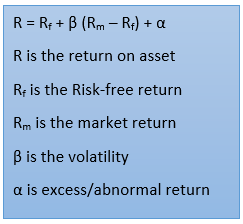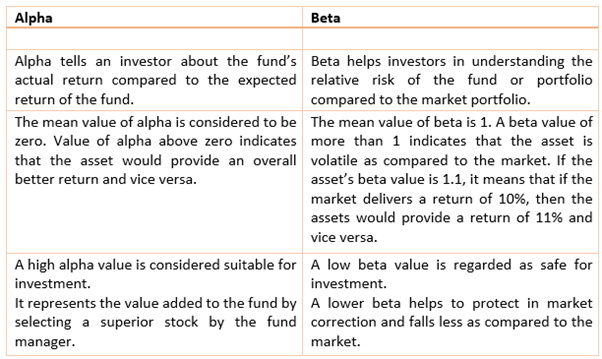# Alpha

• Updated on

## What is alpha?

Alpha is the extra return that an investor earns over and above the benchmark index. Alpha helps in determining the highest possible return with respect to the least amount of risk.The above formula is that of the Capital Asset Pricing Model (CAPM) model, which determines the return an investor needs to realise to balance a particular risk.

What do the terms Alpha and Beta mean in the stock market?

## Simplifying Alpha

We will take an example to understand the concept of alpha. Let us consider that a portfolio manager expects that his/her client’s portfolio would generate a return of 14% next year. However, the actual portfolio return after one year was 16%. In this case, alpha, or excess return, is 2%.

Let us consider another example where an investor gets a return of 20% on his investment on a particular mutual fund while the benchmark index has delivered a return of 18%. It means that the stock has outperformed the benchmark index by 2%. Similarly, if the mutual fund has delivered a return of 18% and the benchmark index has delivered a return of 20%, then the fund has underperformed compared to the benchmark index.

In mutual funds, alpha denotes the performance of the fund manager. In simple words, the higher the value of alpha (α), the better is the fund manager’s performance.

Hence, the higher the alpha value, the higher the chances of the mutual fund delivering improved returns.

It is also important to note that alpha is calculated annually.

## How do we calculate alpha?

From the CAPM formula highlighted above, we can derive the equation of alpha.

α = R – [Rf + β (Rm – Rf)]

1. In the first step of α calculation, it the identification of risk-free rate. It can determine based on the average annual return of government security.
2. In step two, we find the market return. To find the market return, one can track the benchmark index’s annual return over a considerable time frame.
3. In the third step, deduct the risk-free return from the market return. This will give you the value of the risk premium.
4. The fourth step involves assessing the movement of the stock price as compared to the benchmark index. This would return the value of beta.
5. Now calculate the value of the mutual fund or the portfolio or stock using the values calculated in step 1, 2, 3 and 4.
6. Once we get the value of R, Rf, Rm, and β, we place them in the formula of alpha to get the value of alpha.

## What is the significance of alpha?

When we use alpha while making an investment decision, one can see how the fund has performed as compared to the benchmark index. An investor prefers a positive alpha fund instead of a fund with a negative return. A positive alpha value provides an additional return to the overall return on the fund.

For example, if the alpha value is +3 and the fund has delivered a negative return of 10%, then with the positive alpha, the fund’s overall return would be -7% instead of -10%.

## Difference between alpha and beta

Alpha and beta are two standard terms used for measuring investment risk. These are the part of modern portfolio theory. Beta measures the volatility of the asset, while alpha  measures the assets return compared to risk-adjusted expected return.While making any investment decision, alpha should not be the only parameter while selecting a particular fund or stock. One should also look at beta, standard deviation, and Sharpe ratio to make a call.

As alpha helps to determine the performance of the fund manager, a wise investor would do his/her homework and study those funds with higher alpha value. Simultaneously, one must ensure that the fund selected has a consistent track record of having a positive alpha value. This would not only help in understanding the fund manager’s performance but also give an idea of how the fund would do in the future.

##### Top ASX Listed Companies

Definition:
We use cookies to ensure that we give you the best experience on our website. If you continue to use this site we will assume that you are happy with it. OK